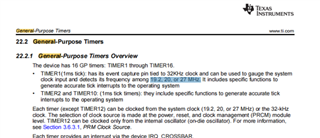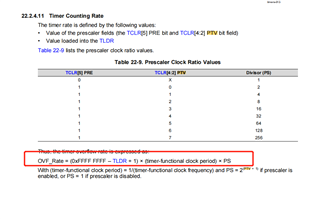If you have a related question, please click the "Ask a related question" button in the top right corner. The newly created question will be automatically linked to this question.

# AM5748: dtimer period issue

Part Number: AM5748

Hello Champs,

RT-linux: ti-processor-sdk-linux-rt-am57xx-evm-05.02.00.10-Linux-x86-Install.bin

Customer tested DMtimer according to below link.

https://e2e.ti.com/support/processors-group/processors/f/processors-forum/415079/dm-timer-beaglebone-black-questions

1. System Clock is 20Mhz, when running below code, the timer is 2s.

priv->timer_ptr = omap_dm_timer_request_by_node(priv->dm_node);

/* Set the Clock source to the SystemClock *
omap_dm_timer_set_source(priv->timer_ptr, OMAP_TIMER_SRC_SYS_CLK);

/* Set prescaler to 1:1 */
omap_dm_timer_set_prescaler(priv->timer_ptr, 0);
/* Determine what IRQ the timer triggers *
priv->timer_irq = omap_dm_timer_get_irq(priv->timer_ptr);

/* Setup the IRQ handler *
ret = request_irq(priv->timer_irq, zhx_timer_irq_handler, IRQF_TIMER, DRIVER_NAME, priv);

system clock is/* Get the Clock rate in Hz */
timer_clk = omap_dm_timer_get_fclk(priv->timer_ptr);
priv->timer_rate = clk_get_rate(timer_clk);
//此时打印出来该数值，priv->timer_rate为20Mhz。

omap_dm_timer_enable(priv->timer_ptr);
omap_dm_timer_set_int_enable(priv->timer_ptr, OMAP_TIMER_INT_OVERFLOW); //使能溢出中断

When timer started, he configured priv->timer_ratio = arg; and then set the initialized value to timer. Timer will count from the initialized value, when it counts to 0x0xffffffff, it will count from the initialized value again.
omap_dm_timer_set_load_start(priv->timer_ptr, 1, 0xffffffff - (priv->timer_ratio * priv->timer_rate));

Question:

The priv->timer_rate from clk_get_rate() is 20M (printed by printk), it means the system clock is 20MHz. But when priv->timer_ratio is 1, 0xffffffff - priv->timer_rate, the timer period should be 1s, why it is still 2s?

Thanks.
Rgds

Shine

• Hi Shine,

The 2 seconds looks correct to me for a priv->timer_ratio value of 1. The timer_rate  value of 20,000,000 in normal operations is 1 second. This is incremented for every 2 cycles if you set and enable the prescaler value of 0 (it is not 1:1). The formula is 2^(value+1), so a prescale value of 0 is equivalent of counter increments every 2 cycles. Please see the GPTimer Register Manual chapter in the TRM, specifically the TCLR.PTV filed description.

What time do you see if you comment out the omap_dm_timer_set_prescale() code?

regards

Suman

• Hi Suman,

Thanks for your help. Customer has resolved his problem by using below red highlighted equation.Rgds
Shine